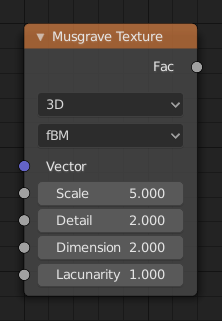# 马氏分形纹理着色器节点¶The Musgrave Texture node evaluates a fractal Perlin noise at the input texture coordinates. Unlike the Noise Texture, which is also a fractal Perlin noise, the Musgrave Texture allows greater control over how octaves are combined.

## 输入选项¶

The inputs are dynamic, they become available if needed depending on the node properties.

Texture coordinate to evaluate the noise at; defaults to Generated texture coordinates if the socket is left unconnected.

W

Texture coordinate to evaluate the noise at.

Scale of the base noise octave.

Number of noise octaves. The fractional part of the input is multiplied by the magnitude of the highest octave. Higher number of octaves corresponds to a higher render time.

The difference between the magnitude of each two consecutive octaves. Larger values corresponds to smaller magnitudes for higher octaves.

The difference between the scale of each two consecutive octaves. Larger values corresponds to larger scale for higher octaves.

An added offset to each octave, determines the level where the highest octave will appear.

An extra multiplier to tune the magnitude of octaves.

## 属性¶

Dimensions

The dimensions of the space to evaluate the noise in.

1D

Evaluate the noise in 1D space at the input W.

2D

Evaluate the noise in 2D space at the input Vector. The Z component is ignored.

3D

Evaluate the noise in 3D space at the input Vector.

4D

Evaluate the noise in 4D space at the input Vector and the input W as the fourth dimension.

Higher dimensions corresponds to higher render time, so lower dimensions should be used unless higher dimensions are necessary.

Type of the Musgrave texture.

fBM (分形布朗运动)

Height

Texture value.